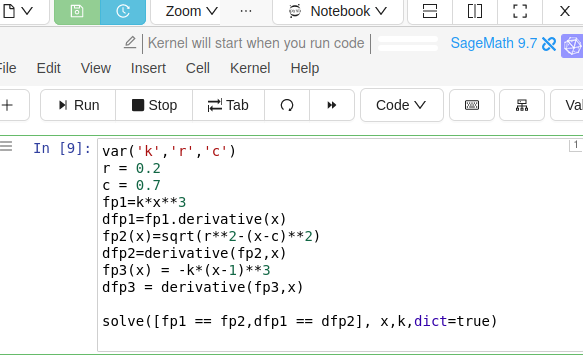# Revision history [back]

### solving 2 non-linear functions (max degree of 3) fails

I'm having trouble solving the following:

var('k','r','c') r = 0.2 c = 0.7 fp1=kx3 dfp1=fp1.derivative(x) fp2(x)=sqrt(r2-(x-c)2) dfp2=derivative(fp2,x) fp3(x) = -k(x-1)3 dfp3 = derivative(fp3,x)

solve([fp1 == fp2,dfp1 == dfp2], x,k,dict=true)

It's a simple system that uses the functions and their respective derivatives to find values fo x and k. Mathematica has no problem with this. My machine and the cocalc machine become compute bound. My machine runs out of memory. How do I redo this to get sage to solve it ? Should I try a numeric solve ?

### solving 2 non-linear functions (max degree of 3) fails

I'm having trouble solving the following:

var('k','r','c') r = 0.2 c = 0.7 fp1=kx3 dfp1=fp1.derivative(x) fp2(x)=sqrt(r2-(x-c)2) dfp2=derivative(fp2,x) fp3(x) = -k(x-1)3 dfp3 = derivative(fp3,x)

solve([fp1 == fp2,dfp1 == dfp2], x,k,dict=true)It's a simple system that uses the functions and their respective derivatives to find values fo x and k. Mathematica has no problem with this. My machine and the cocalc machine become compute bound. My machine runs out of memory. How do I redo this to get sage to solve it ? Should I try a numeric solve ?

### solving 2 non-linear functions (max degree of 3) fails

I'm having trouble solving the following:

var('k','r','c') r = 0.2 c = 0.7 fp1=kx3 dfp1=fp1.derivative(x) fp2(x)=sqrt(r2-(x-c)2) dfp2=derivative(fp2,x) fp3(x) = -k(x-1)3 dfp3 = derivative(fp3,x)

solve([fp1 == fp2,dfp1 == dfp2], x,k,dict=true)It's a simple system that uses the functions and their respective derivatives to find values fo x and k. Mathematica has no problem with this. My machine and the cocalc machine become compute bound. My machine runs out of memory. How do I redo this to get sage to solve it ? Should I try a numeric solve ?